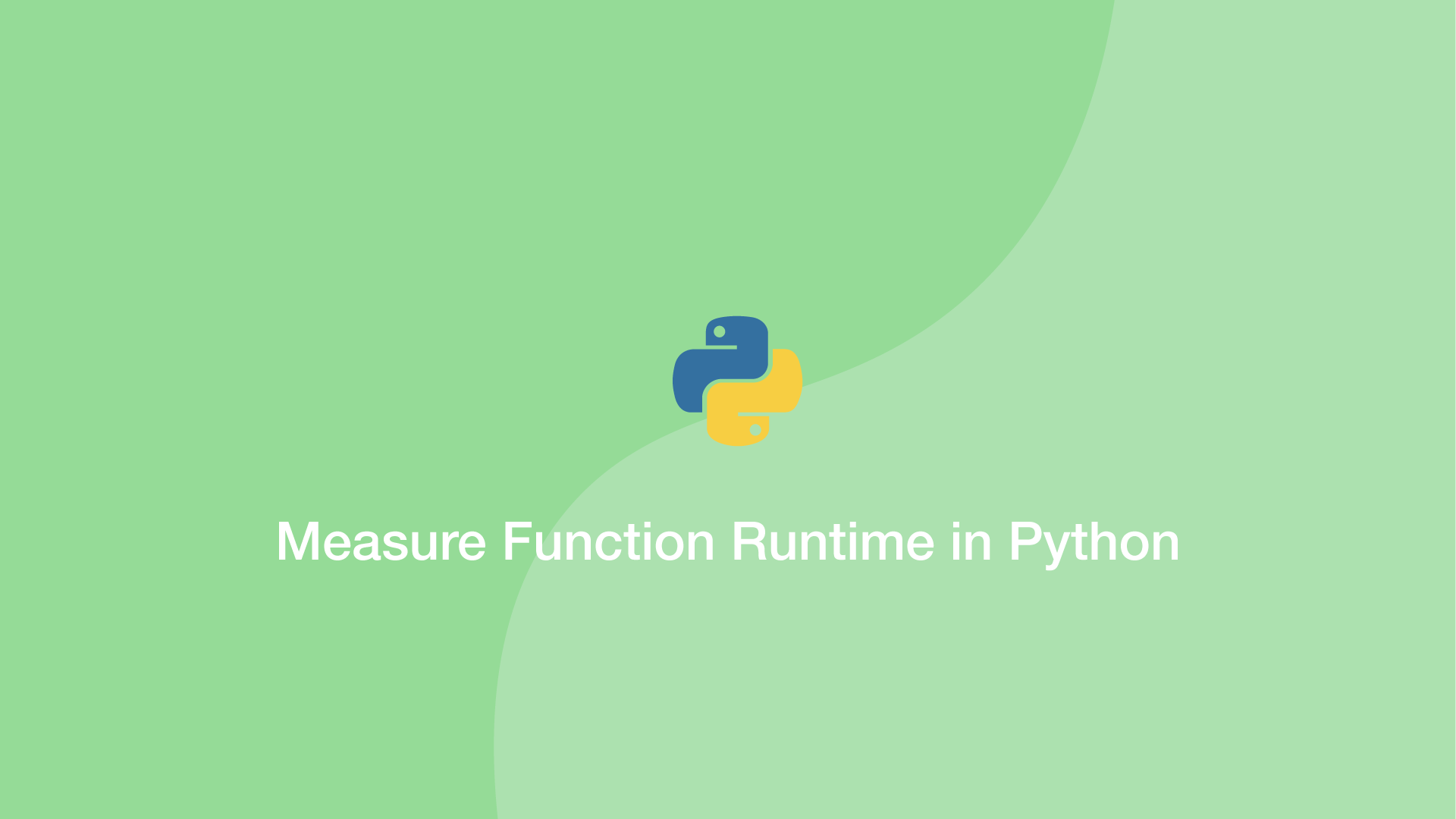# How to Measure Function Runtime in PythonIn this tutorial, we will learn how to create timer functions in Python to measure the performance of any function in a program.

To do this, we will make use of the Python `time` package. This will allow us to create Unix timestamps at the start and end of a function that we can compare to deduce runtime.

Let's look at an example timer function and break it down.

``````import time

start = time.time()

for r in range(1,40000000):
pass

end = time.time()

print(format(end - start))
``````
``````1.2815041542053223
``````

In the above example we generate a new Unix timestamp before the code to test using `time.time()` and store it in a variable called `start` – the same is done after the code and stored in the variable `end`.

Then we subtract the timestamps to get the difference in seconds. In the above case, it took Python 1.28 seconds to loop 40 million times.

#### Related Tutorials### How to use setInterval() in JavaScript

June 02, 2021### How to Create and Use Lists (Arrays) in Python

September 01, 2020### How To Use Functions in Python

August 31, 2020### How to Calculate Mean, Median, Mode and Range in Python

October 07, 2020### How to Use Classes and Objects in Python

September 15, 2020### How to use the Python filter() Function

September 28, 2020